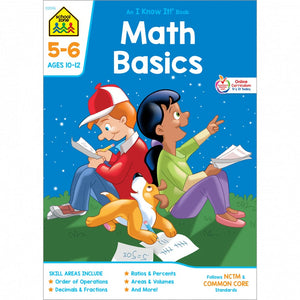School Zone Math Basics Grades 5-6 Workbook

Regular price \$3.99 \$0.00 Unit price per

Build a solid foundation for higher math success! The Math Basics 5-6 workbook teaches and reinforces the math concepts that fifth and sixth graders need to practice the most before moving on to junior high-level hurdles. Interesting, real-world activities and examples introduce and reinforce important skills such as decimals, estimating, multiplication, fractions, averages, percents and more. End-of-page brain bogglers and challenge questions add to the fun and learning.The workbook is aligned with the Common Core State Standards for Mathematics, a comprehensive and progressive set of learning objectives created to help students succeed in math. It is also consistent with the Principles and Standards for School Mathematics, a publication by the National Council of Teachers of Mathematics (NCTM), and it is compatible with Singapore math pedagogy. The workbook provides the highest quality, most up-to-date curriculum possible. For a well-rounded math education, get the School Zone Advantage!

Skills

• place value
• whole numbers
• decimals
• multiplying and dividing decimals
• averages
• order of operations
• solving equations
• fractions